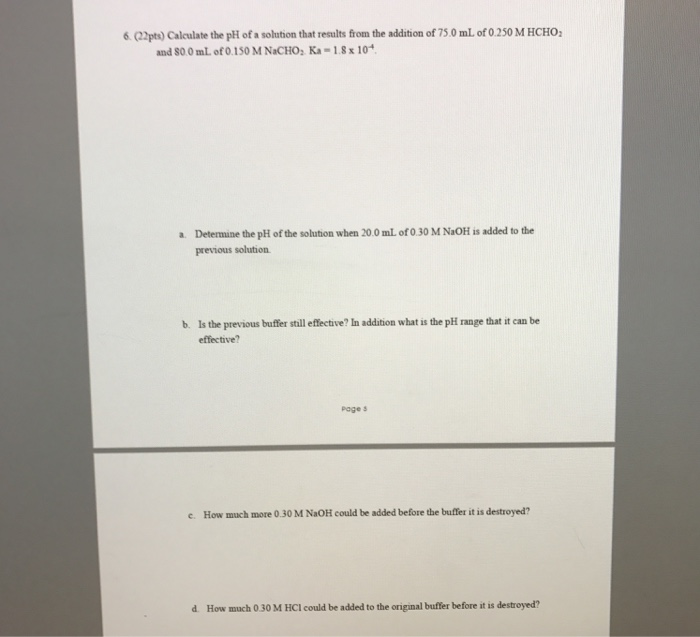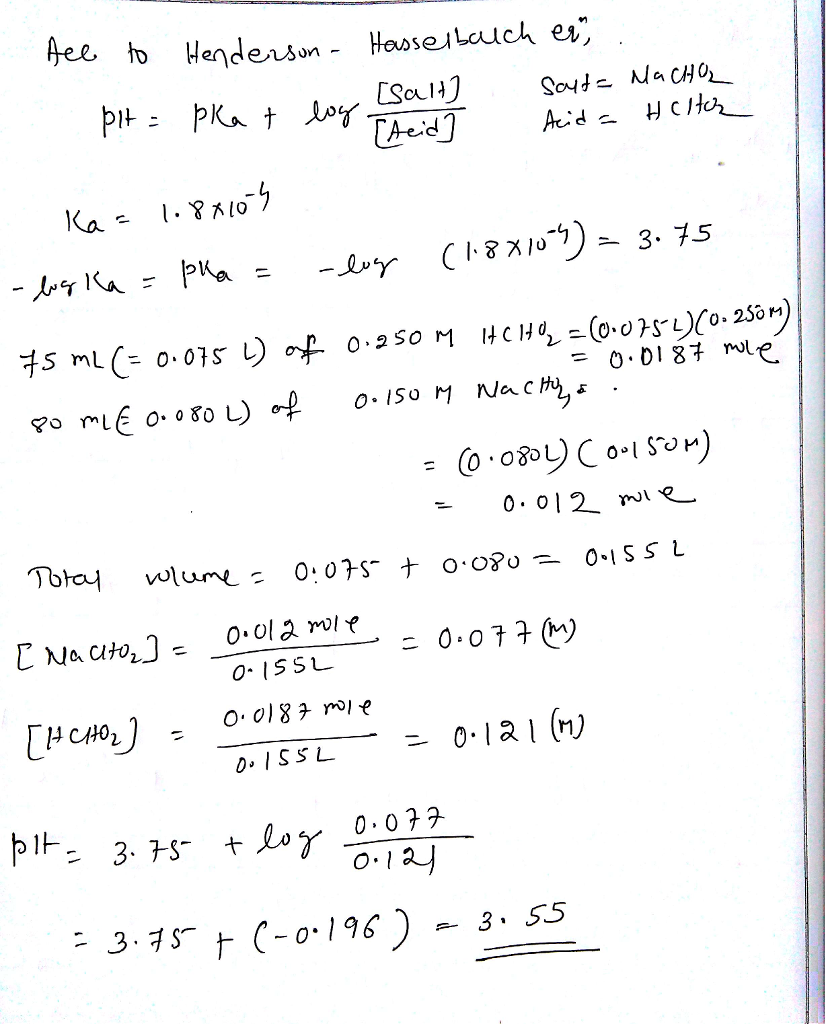# Question & Answer: Calculate the pH of a solution that results from the addition of 75.0 mL of 0.250 M HCHO_2…..Please do it step by step and clear

Calculate the pH of a solution that results from the addition of 75.0 mL of 0.250 M HCHO_2 and 80.0 mL of 0.150 M NaCHO_2. Ka = 1.8 times 10^-4. a. Determine the pH of the solution when 20.0 mL of 0.30 M NaOH is added to the previous solution. b. Is the previous buffer still effective? In addition what is the pH range that it can be effective? c. How much more 0.30 M NaOH could be added before the buffer it is destroyed? d. How much 0.30 M HCl could be added to the original buffer before it is destroyed?